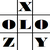anything in Excel...

It's Excel-o-lozy...

### Excel vLookup formula Limitations are resolved.. under formulaFix by Excelolozy.

www.Excelolozy.com - "avLookup" is the formula with advanced features of vLookup.

vLookup in Excel has a limitation to search the data from first column only.

"avLookup" has following 2 features :
• It can search from any column
• Search column can exist even after the Result column.

The formula is :
=avLookup(LookupValue, RangeRef, SearchColumn, ResultColumn, ExactMatch)

Where

• LookupValue - Value to be searched
• RangeRef - Table reference
• SearchColumn - Number of Column where above value is to be searched from
- It's optional, can be omitted, by default it is 1
- i.e. it'll search 1st column of table if nothing entered
• ResultColumn - Number of Column where result is to be picked from
- It's optional, can be omitted, by default it is 2
- i.e. it'll search 2nd column of table if nothing entered
• ExactMatch - 0 means Exact value to be searched, 1 is for nearest value
- It's optional, can be omitted, by default it is 0
- i.e. it'll search Exact match if nothing entered

Magic is that search Column can be after Result Column.

The formula "Comment" is available within tool named "formulaFix".

"formulaFix" is a "Formula (fx) Fixing Tool" that provides various formulas that don't exist in Excel.